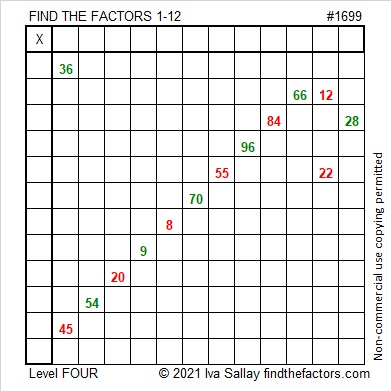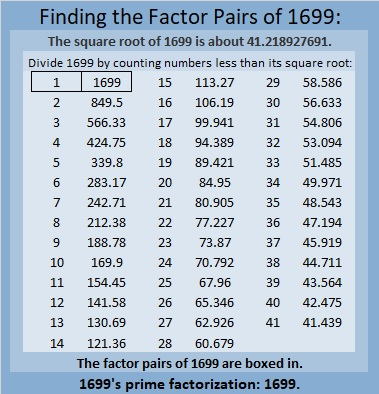# 1699 Sweet Candy Cane

Contents

### Today’s Puzzle:

Solving this candy cane puzzle can be a sweet experience. Just use logic to write the numbers 1 to 12 in both the first column and the top row so that those numbers and the given clues make a multiplication table.### Factors of 1699:

• 1699 is a prime number.
• Prime factorization: 1699 is prime.
• 1699 has no exponents greater than 1 in its prime factorization, so √1699 cannot be simplified.
• The exponent in the prime factorization is 1. Adding one to that exponent we get (1 + 1) = 2. Therefore 1699 has exactly 2 factors.
• The factors of 1699 are outlined with their factor pair partners in the graphic below.How do we know that 1699 is a prime number? If 1699 were not a prime number, then it would be divisible by at least one prime number less than or equal to √1699. Since 1699 cannot be divided evenly by 2, 3, 5, 7, 11, 13, 17, 19, 23, 29, 31, 37, or 41, we know that 1699 is a prime number.

### More About the Number 1699:

1699 is the third prime in a prime triple. What are the other two primes in that triple?

1699 is the difference of two squares:
850² – 849² = 1699.

This site uses Akismet to reduce spam. Learn how your comment data is processed.